# Study on penetration characteristics of honeycomb aluminum

## Wenbin Du1, Hongxiao Chao2, Qiang Lei3, Junlong Ren4, Dongyin Wang5

1, 2, 3, 4, 5Northwest Institute of Mechanical and Electrical Engineering, 712099, Xianyang, P. R. China

3Corresponding author

Vibroengineering PROCEDIA, Vol. 28, 2019, p. 160-164. https://doi.org/10.21595/vp.2019.21035
Received 19 September 2019; accepted 29 September 2019; published 19 October 2019

42nd International Conference on Vibroengineering in Shanghai, China, October 19-21, 2019

Copyright © 2019 Wenbin Du, et al. This is an open access article distributed under the Creative Commons Attribution License, which permits unrestricted use, distribution, and reproduction in any medium, provided the original work is properly cited.
Views 46
CrossRef Citations 0
Abstract.

In order to analyze the mechanical properties of projectile penetrating honeycomb aluminum, a dynamic nonlinear finite element model of projectile penetrating honeycomb aluminum was established and verified by experiments. It is found that the numerical simulation results of projectile penetration into honeycomb aluminum are consistent with the acceleration variation of the test. When the projectile penetrates into the honeycomb aluminum, the acceleration waveform is trapezoidal and the peak and pulse width of the acceleration are related to the velocity of the projectile and the wall thickness of the honeycomb aluminum material. With the increase of the velocity of the projectile, the peak value of the acceleration increases and the pulse width of the acceleration decreases. With the increase of the wall thickness of honeycomb aluminum, the peak value of acceleration increases, while the pulse width of acceleration decreases.

Highlights
• Simulation method can effectively analyze the penetration characteristics of honeycomb aluminum.
• The peak acceleration increases and the acceleration pulse width increases with the increase of projectile velocity.
• The peak acceleration increases and the acceleration pulse width decreases with the increase of honeycomb aluminum wall thickness.
• Simulation method can be used to study the penetration characteristic of honeycomb aluminum.

Keywords: honeycomb aluminum, penetration, acceleration, simulation.

#### 1. Introduction

Honeycomb aluminum is a good cushioning energy absorption material, which can absorb a lot of energy in the process of dynamic impact, so it is made into a variety of cushioning energy absorption components, which are widely used in military, transportation, packaging, construction and other fields.

The coplanar and heteroplanar mechanical properties of honeycomb materials were analyzed theoretically by Gibson and Ashby . Yu Jiang et al.  simulated and studied the coplanar impact mechanical properties of circular honeycomb aluminum. D. Ruan et al.  studied the deformation mode and platform stress of honeycomb aluminum under in-plane dynamic compression by finite element analysis. Sun Deqiang et al. . studied the deformation mode, elastic modulus and energy absorption of double-walled thick honeycomb aluminum core under coplanar impact load. Hul L. et al. . studied the in-plane collapse deformation mechanism of honeycomb aluminum under high speed impact by LS-DYNA, and gave the platform stress formula of honeycomb aluminum under dynamic load. Zou Z. et al.  studied the platform stress and energy absorption of honeycomb aluminum under coplanar impact load by numerical simulation. The in-plane quasi-static platform stress of honeycomb is analyzed by Qiu X. M.  theory, the in-plane dynamic response of honeycomb aluminum is simulated, and the platform stress formula under dynamic load is obtained. T. Wierzbicki et al.  studied the compressive mechanical properties of metal honeycomb on different surfaces, and put forward the formula of static collapse stress on different surfaces. M. Yamashita  analyzed the out-of-plane compression mechanical behavior of honeycomb aluminum by means of experiment and numerical simulation.

Most of the above studies are about the compressive mechanical properties of honeycomb aluminum, and the penetration of honeycomb aluminum is rarely involved. Therefore, in this paper, the mechanical behavior of projectile penetrating into honeycomb aluminum with different wall thickness is studied by means of numerical simulation and experiment.

#### 2. Simulation and analysis of projectile penetrating honeycomb aluminum

The shell element finite element model of projectile penetrating honeycomb aluminum is established by using LS-DYNA. In order to reduce the calculation scale, according to the symmetry, the model is established, as shown in Fig. 1.

Fig. 1. Model of projectile penetrating into honeycomb aluminum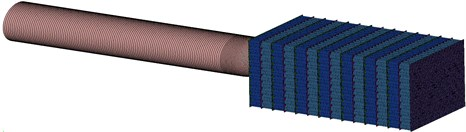The projectile is a spherical projectile with a warhead radius of 55 mm, a cylinder and a hollow structure. In this paper, ten layers of stacked honeycomb aluminum plate are studied in this paper. The specification of each plate is 200 mm × 200 mm × 50 mm, and the honeycomb aluminum is commercial double-wall thick honeycomb aluminum. The related simulation parameters as shown in Table 1 and Table 2.

Table 1. Simulation parameters of projectile material

 Material brand Density Elastic modulus Poisson’s ratio 2A11 2.79 g/cm3 68 GPa 0.33

Table 2. Simulation parameters of honeycomb aluminum material

 Wall thickness / t Size Density Elastic modulus Poisson’s ratio 0.2 mm 200×200×50 2700 g/cm3 68.9 MPa 0.35 0.3 mm 200×200×50 2700 g/cm3 68.9 MPa 0.35

#### 3. Experiment of projectile penetrating honeycomb aluminum

The air gun is used as the power device for the projectile to penetrate the honeycomb aluminum. The experiment system is composed as shown in Fig. 2. The projectile is mounted inside the barrel, close to the high pressure chamber. When launched, the release mechanism opens quickly, the gas pressure is applied to the projectile, and the projectile is accelerated until it flies out of the muzzle. In the process of penetrating into honeycomb aluminum, the local fracture and compression of honeycomb aluminum are caused by projectile. The penetration displacement of projectile is measured by high-speed camera and motion analysis software. The impact acceleration is obtained by quadratic differential operation after data preprocessing.

Fig. 2. The experiment system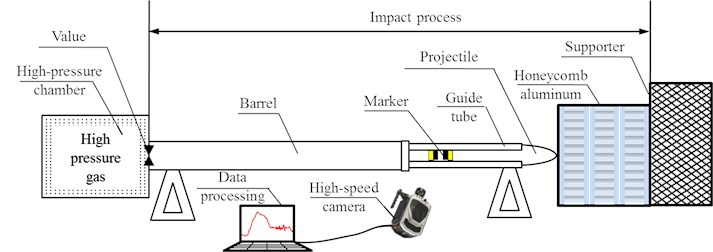#### 4.1. Results of simulation and experiment

The deformation of honeycomb aluminum and finite element simulation after the experiment is shown in Fig. 3. It can be seen from Fig. 3 that the projectile penetrates into the honeycomb aluminum mainly due to local shearing and tearing deformation, and the overall structure deformation of the honeycomb aluminum is small. This is because the strength of the experiment honeycomb aluminum is low, and the honeycomb aluminum is first broken and destroyed when it is impact by the projectile, and the overall structure is not subjected to sufficient force to deform it.

Fig. 3. Simulation and experimental results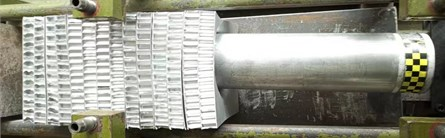a) Experiment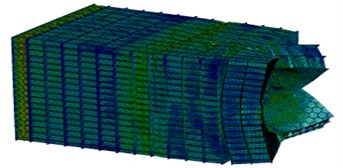b) Simulation

#### 4.2. Discussion of simulation and experimental results

(1) Relationship between projectile velocity and acceleration.

The acceleration curve of the projectile at different velocity is shown in Fig. 4(a), and the peak acceleration and pulse width (acceleration duration) are shown in Table 3. The results show that with the increase of projectile velocity, the initial kinetic energy obtained by projectile increases, the peak acceleration increases, and the pulse width of acceleration increases. In addition, with the increase of projectile velocity, the velocity of projectile acceleration reaching the maximum peak is faster.

Fig. 4. a) Acceleration of projectiles with different impact velocities (0.3 mm), b) different wall thickness projectile acceleration curves (130 m/s)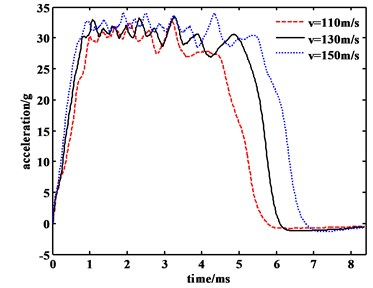a)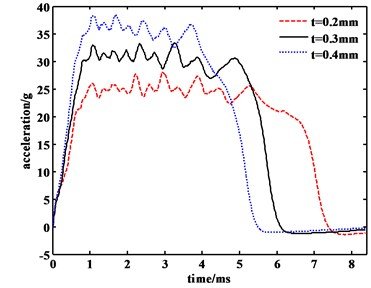b)

Table 3. Impact acceleration peak and pulse width of projectiles with different impact velocities

 Velocity (m/s) Acceleration peak (g) Pulse width (ms) 110 3260 5.83 130 3276 6.18 150 3387 6.96

#### 5. Relationship between wall thickness and acceleration

The acceleration curve of the projectile at different wall thickness is shown in Fig. 4(b), and the peak acceleration and pulse width (acceleration duration) are shown in Table 4. The results show that with the increase of wall thickness, the relative stiffness of honeycomb aluminum increases, the peak acceleration increases, and the acceleration pulse width decreases. In addition, with the increase of wall thickness, the velocity of projectile acceleration reaching the maximum peak is faster.

Table 4. Impact acceleration peak and pulse width of projectiles with different impact velocities

 Wall thickness (mm) Acceleration peak (g) Pulse width (ms) 0.2 2964 7.57 0.3 3276 6.18 0.4 3819 5.59

#### 5.1. Comparison between simulation and experiment

By comparing the simulation results and experimental results can make a mutual authentication between the two methods. The results are shown in the Table 5. As it can be seen the simulation results can be good fit with the experiment results. So in the next study, we can use the simulation to study its penetration characteristic.

Table 5. Comparison between simulation and experiment

 Projectile velocity (m/s) Wall thickness (mm) Item Acceleration peak (g) Pulse width (ms) 110 0.2 Simulation 2952 6.20 Experiment 2950 6.21 Error -2 0.01 0.3 Simulation 3261 5.85 Experiment 3260 5.83 Error –1 –0.02 0.4 Simulation 3842 5.30 Experiment 3845 5.24 Error 3 –0.06 130 0.2 Simulation 2966 7.62 Experiment 2964 7.57 Error -2 0.05 0.3 Simulation 3271 6.27 Experiment 3276 6.18 Error 5 –0.09 0.4 Simulation 3810 5.32 Experiment 3819 5.59 Error -9 0.27 150 0.2 Simulation 3349 8.11 Experiment 3358 8.32 Error -9 0.21 0.3 Simulation 3385 6.98 Experiment 3387 6.96 Error 2 –0.02 0.4 Simulation 3935 6.20 Experiment 3942 6.39 Error –7 0.19

#### 6. Conclusions

First, simulation method can effectively analyze the penetration characteristics of honeycomb aluminum. Second, with the increase of projectile velocity, the peak acceleration increases and the acceleration pulse width increases. Third, with the increase of honeycomb aluminum wall thickness, the peak acceleration increases and the acceleration pulse width decreases. Forth, simulation method can be used to study the penetration characteristic of honeycomb aluminum and compared with experiment it has some advantages; it can makes a further optimization analysis of the structure of honeycomb aluminum.

1. Gibson L. J., Ashby M. F. Cellular Solids: Structure and Properties. Pergamon Press, Oxford, 1988. [Search CrossRef]
2. Yu Jiang, Li Xiao-Juan Finite element analysis on the impact performance of circular metal honeycomb Core. Packaging Engineering, Vol. 29, Issue 11, 2008, p. 30-31. [Search CrossRef]
3. Ruan D., Lu G., Wang B., et al. In-plane dynamic crushing of honeycombs: a finite element study. International Journal of Impact Engineering, Vol. 28, Issue 3, 2003, p. 161-182. [Publisher]
4. Sun De-Qiang, Zhang Wei-Hong In-plane impact properties of aluminum double-walled honeycomb cores. Journal of Vibration and Shock, Vol. 27, Issue 7, 2008, p. 69-74. [Search CrossRef]
5. Hu L. L., Yu T. X. Dynamic crushing strength of hexagonal honeycombs. International Journal of Impact Engineering, Vol. 37, 2010, p. 467-474. [Publisher]
6. Zou Z., Reid S. R., Tan P. J., et al. Dynamic crushing of honeycombs and features of shock fronts. International Journal of Impact Engineering, Vol. 36, 2009, p. 165-176. [Publisher]
7. Qiu X. M., Zhang J., Yu T. X. Collapse of periodic planar lattices under uniaxial compression, part I: quasi-static strength predicted by limit analysis. International Journal of Impact Engineering, Vol. 36, 2009, p. 1223-1230. [Publisher]
8. Wierzbicki T. Crushing analysis of metal honeycombs. International Journal of Impact Engineering, Vol. 1, Issue 2, 1983, p. 157-174. [Publisher]
9. Yamashita M., Gotoh M. Impact behavior of honeycomb structures with various cell specifications-numerical simulation and experiment. International Journal of Impact Engineering, Vol. 32, Issues 1-4, 2005, p. 618-630. [Publisher]